## Rutherford-Geiger-Marsden (Gold Foil) Experiment

In this experiment, positively charged aalpha particles were directed at gold foil with a fluorescent screen to detect the alpha particles after they interacted with the foil.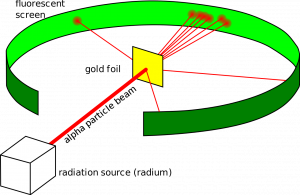Most of the alpha particles passed straight through the foil, with others passing through on a deflected path and a smaller amount bouncing back off the foil.

This led to a number of conclusions:

1. Most of the atom is empty space (because most alpha particles passed through)
2. Most of the mass is concentrated in a small space (nucleus)
3. Nucleus has a positive charge (because it repelled the positive alpha particles)
4. Nucleus is surrounded by orbiting electrons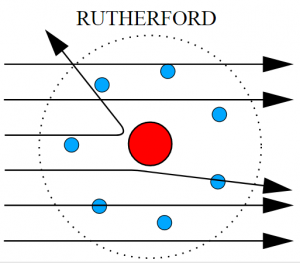## Scientific Method

The scientific method describes how theories are developed and modified:

1. Evidence is collected from an experiment
2. A theory is developed to explain the evidence
3. The theory is tested with further investigations/results
4. The theory is modified if necessary based on the further evidence

You use this process regularly without realising it! For example:

1. Light bulb does not come on when you press the switch (evidence)
2. The bulb might be broken (theory)
3. Replace bulb and try again (testing theory)
4. If new bulb works, your theory was proved to be correct
5. If new bulb does not work, your theory needs to be modified (eg there may be a problem with the wiring)

## Isotopes

The atomic number for an element is the number of protons in the nucleus of that element. This does not change for a particular element. If there are a different number of protons it is a different element.

The mass number is the number of particles in the nucleus (protons and neutrons) for an element. The mass number for a particular element can vary as the number of neutrons can be different (still the same element).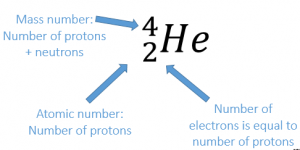Isotopes have the same number of protons as the original element but different numbers of neutrons.

Some isotopes are unstable/radioactive. These unstable isotopes will decay, emitting radiation from the nucleus.

Hydrogen

For example, most hydrogen atoms have 1 proton and 1 electron: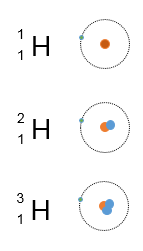But two other isotopes of hydrogen also exist:

Deuterium (1 proton and 1 neutron)

Tritium (1 proton and 2 neutrons)

Carbon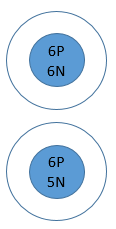Most Carbon exists as Carbon-12 (6 protons & 6 neutrons)

Carbon-11 is an isotope of carbon that has 6 protons (because carbon always has 6 protons) and 5 neutrons (because the mass number is 11)

Other isotopes of carbon also exist. Some isotopes are unstable (radioactive).

For isotopes:

1. Mass number is different
2. Atomic number is the same

When an unstable isotope decays, it releases radiation from the nucleus. The remaining nucleus will have different numbers of particles.

There are three types of radiation – alpha, beta and gamma: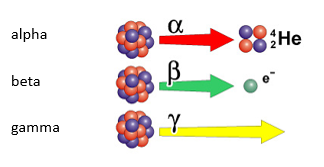## Developing the Model of the Atom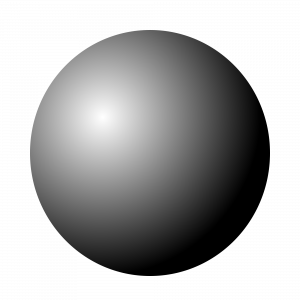1. The Greek philosopher Democritus named the smallest (indivisible) parts of a substance ‘atoms’
2. In 1897 electrons were discovered. This led to the ‘plum pudding’ model of the atom – that negative electrons were spread throughout a positive sphere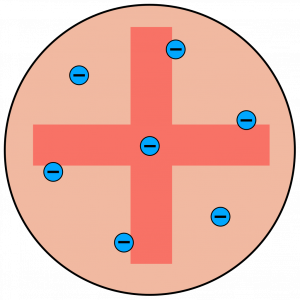1. In 1911 the gold foil experiment discovered the existence of a nucleus and that most of the atom is empty space. This led to the nuclear model of the atom – Atoms have a nucleus with orbiting electrons
2. In 1932 this was further modified to define protons and neutrons within the nucleus.

You are not expected to remember these dates or details. You do need to be familiar with the fact that the model of the atom has been developed over time in response to experimental results.

## Alpha

Alpha radiation is made up of 2 protons and 2 neutrons (Helium Nucleus).When alpha radiation is released from a nucleus, the remaining nucleus will be smaller.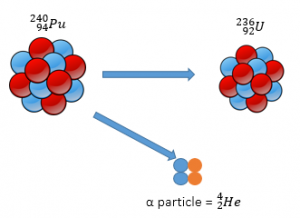Alpha Decay Equation

Alpha decay can be represented using an equation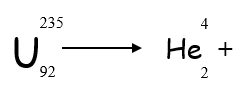To work out what element is left after the decay:

1. Balance mass numbers on each side of the equation
2. Balance atomic numbers on each side of the equation
3. Look up ‘daughter’ element in periodic table __using atomic number __(NOT mass number as isotopes can have different mass numbers)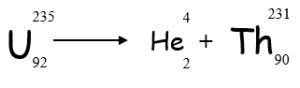## Beta

With Beta decay, a neutron decays into a proton and an electron. The proton remains in the nucleus and the electron is released. (Beta radiation is an electron)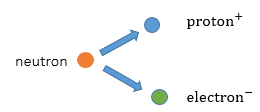The remaining nucleus will have the same mass but will have one more proton and one less neutron.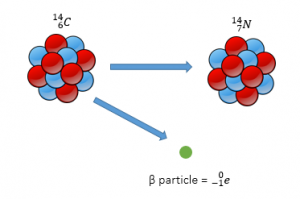Beta Decay Equation

Beta decay can also be represented using an equation: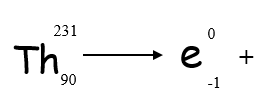1. Balance mass numbers on each side of the equation
2. Balance atomic numbers on each side of the equation (one extra proton in ‘daughter’ element)
3. Look up ‘daughter’ element in periodic table __using atomic number __(NOT mass number as isotopes can have different mass numbers)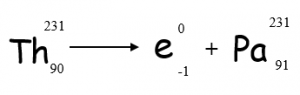## Gamma

Gamma is an electromagnetic wave. As it is not a particle, it has no mass.

It does not affect the number of particles or mass of the nucleus, so there is no decay equation for gamma radiation.

How many neutrons are in the nucleus of an atom of Oxygen-16?
8
How many neutrons are in the nucleus of an atom of Oxygen-14?
6
If Potassium-40 decays by emitting beta radiation, what is the mass number of the remaining isotope?
40
If Uranium-235 decays by emitting alpha radiation, what is the mass number of the remaining isotope?
231